Course Content

0/1

0/3

0/5

0/3

0/2

0/4

0/2

0/1

0/5

5.3 Ellipse 椭圆

1. Find the centre, vertex, axis of symmetry, major semi-axis, minor semi-axis, eccentricity, focus, directrix and length of latus rectum for the following ellipse and sketch the graph.

(a)(b)(c)(d)(e)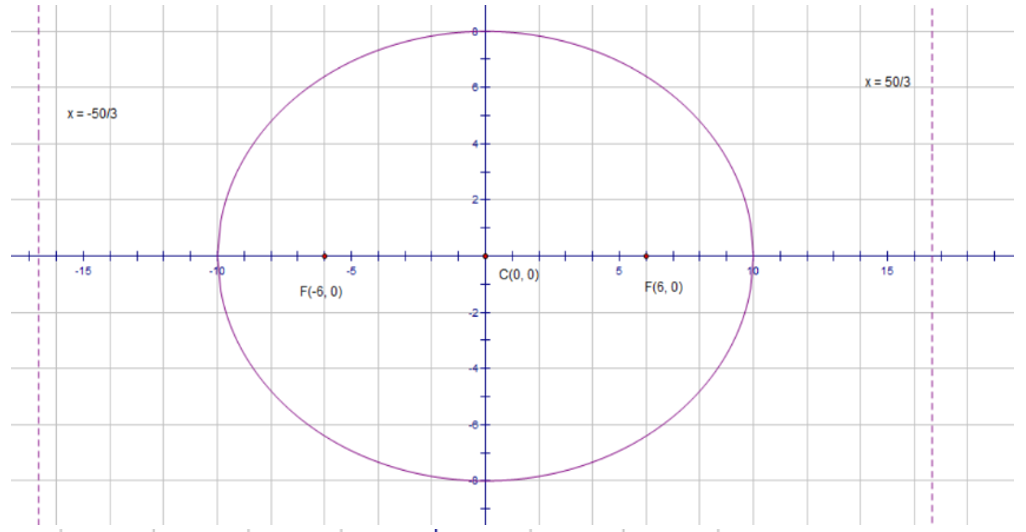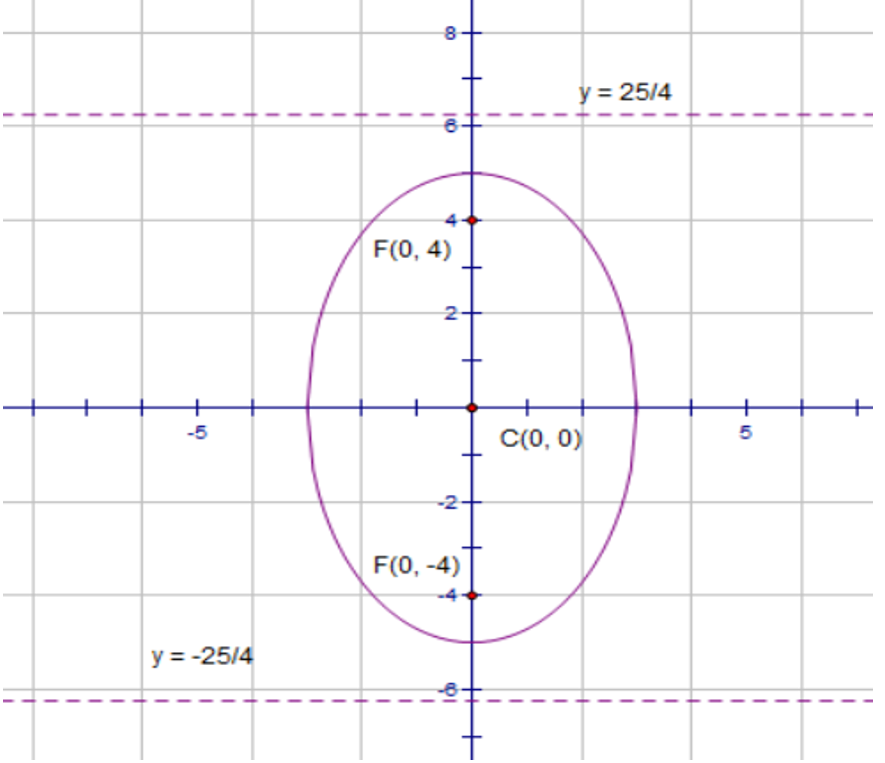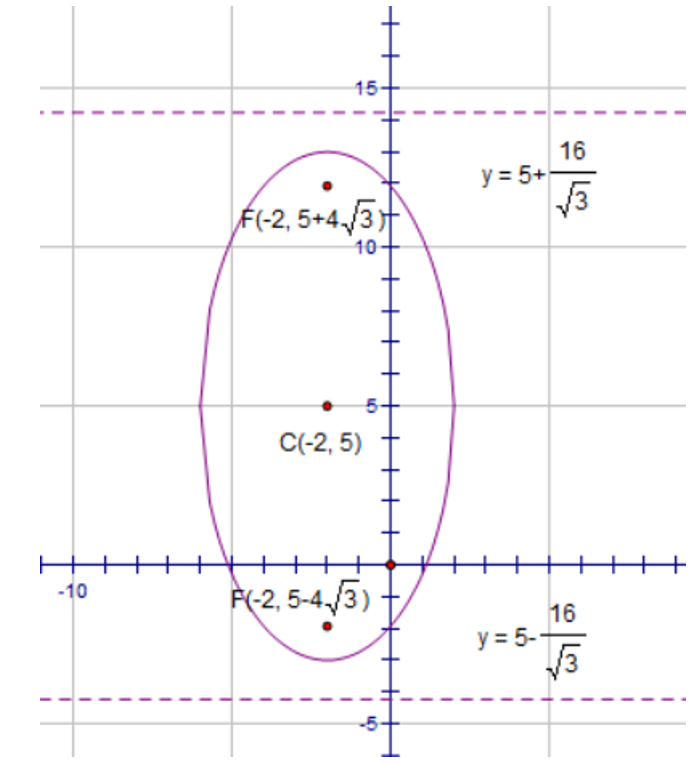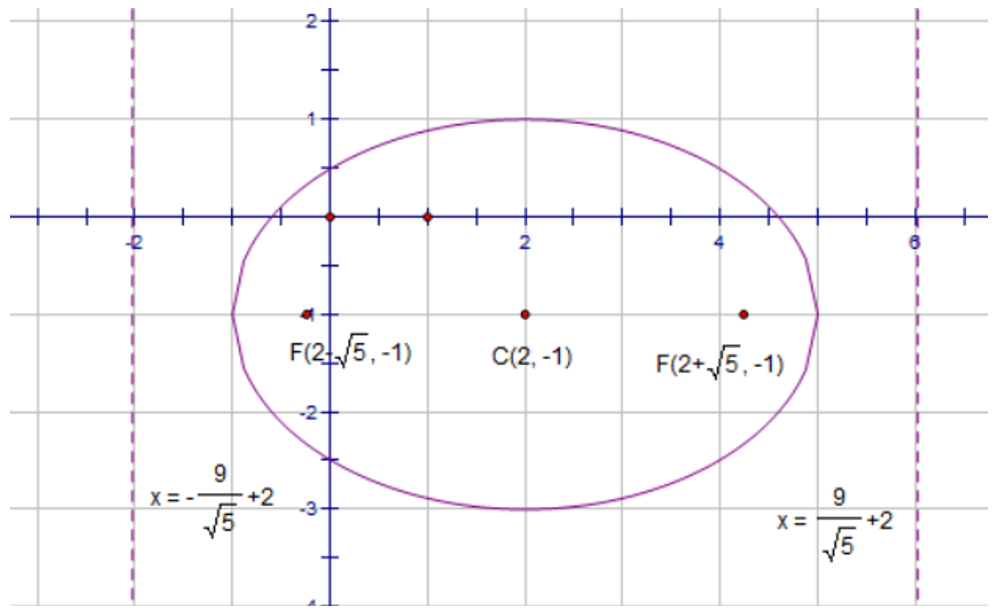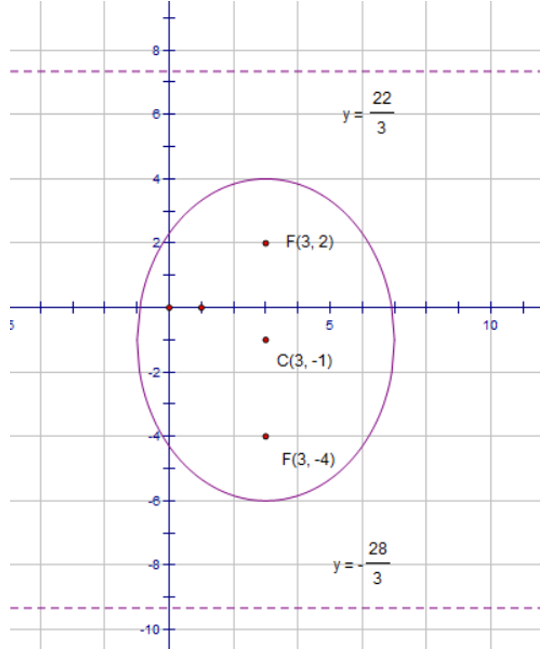2. Find the equation of the ellipse with center at (-3, 1), one of vertices at (-5, 1), length of latus rectum equal to 1 and

(a) major axis parallel x-axis,

(b) major axis parallel y-axis,

(a)(b)3. Find the equation of the ellipse which has same eccentricity and left directrix with ellipse, and also take its right focus to be the left focus.4. Given that the major axis of an ellipse is 4, y-axis be its directrix, the left focus is on the parabola. Find the equation of the ellipse when eccentricity is.5. Given that F(2, -2), F(2, 0) are the foci of a ellipse, straight line y = 3 is a directrix of the ellipse, find the equation of the ellipse.6. Find the equation of the ellipse with vertices at (0, -1) and (12, -1), a focus at.